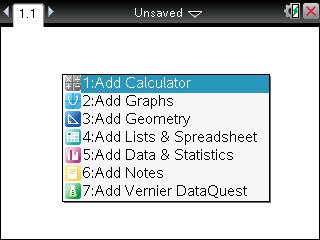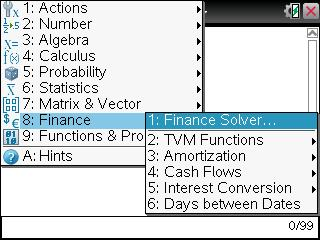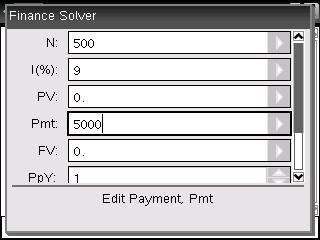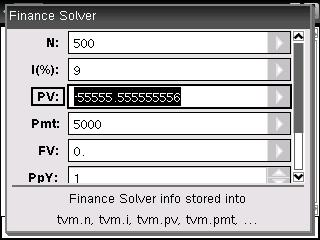# Knowledge Base

## Solution 28130: Calculating the Present Value of a Perpetuity (Infinite Annuity) on the TI-Nspire™ Family.

### How can I calculate the present value of a perpetuity (infinite annuity) on a TI-Nspire family handheld or software?

Sometimes when dealing with annuities, there are those that pay forever instead of for a specific period of time. Hence, they are called perpetuities or infinite annuities. Since these units do not have a way to specify an infinite number of periods for N, the calculation involves the fact that the present value of a cash flow far enough into the future is going to be approximately $0. This means that futuristically the cash flows no longer add anything to the present value. Therefore, selecting a large number of payments yields a close approximation to a perpetuity. Example: The payment is$5,000 per year and the interest rate is 9% annually. Since it is a perpetuity, the user can select 500 as N.

1) Access the TVM solver by opening a calculator page.Once the calculator page is open press [Menu] and select 8:Finance. From the Finance menu select option 1:Finance Solver.2) Input the following:Please Note: The term N has to be a very large number.

3) Place the cursor next to PV by pressing [tab] and press [enter] to compute the present value.

The present value is \$55,555.56Please Note: There is not a way to find the future value of a perpetuity because the cash flows never end.